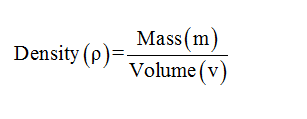# Compute the density in g/cm3 of a piece of metal that has a mass of 0.500 kg and a volume of 63 cm3.

Question
70 views

Compute the density in g/cm3 of a piece of metal that has a mass of 0.500 kg and a volume of 63 cm3.

check_circle

Step 1

Given :

Mass(m)=0.500Kg=500gm.

Volume(v)=63cm3

Step 2

Formula we use:Step 3

Calculatio...

### Want to see the full answer?

See Solution

#### Want to see this answer and more?

Solutions are written by subject experts who are available 24/7. Questions are typically answered within 1 hour.*

See Solution
*Response times may vary by subject and question.
Tagged in

### Physics